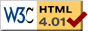### Journée-séminaire de combinatoire

#### (équipe CALIN du LIPN, université Paris-Nord, Villetaneuse)

Le 23 octobre 2012 à 14h00 en B107, Juanjo Rué Perna nous parlera de : On the probability of planarity of a random graph near the critical point

Résumé : Consider the uniform random graph G(n,M) with n vertices and M edges. Erdős and Rényi (1960) conjectured that when n goes to infinity, then the limit of "Pr(G(n,n/2) is planar" exists and is a constant strictly between 0 and 1. Łuczak, Pittel and Wierman (1994) proved this conjecture and Janson, Łuczak, Knuth and Pittel (1993) gave lower and upper bounds for this probability. In this talk we show how to determine the exact probability of a random graph beingplanar near the critical point M=n/2. More exactly, for each λ, we find an exact analytic expression for p(λ) = \lim_{n \to \infty} \Pr{G(n,n/2 (1+λ n^{-1/3})) is planar}. In particular, we obtain p(0) \approx 0.99780. If time permits we will show extensions of these techniques and further research. This is joint work with Marc Noy (UPC-Barcelona) and Vlady Ravelomanana (LIAFA-Paris)

 Dernière modification : Monday 24 January 2022Contact pour cette page : Cyril.Banderier at lipn.univ-paris13.fr